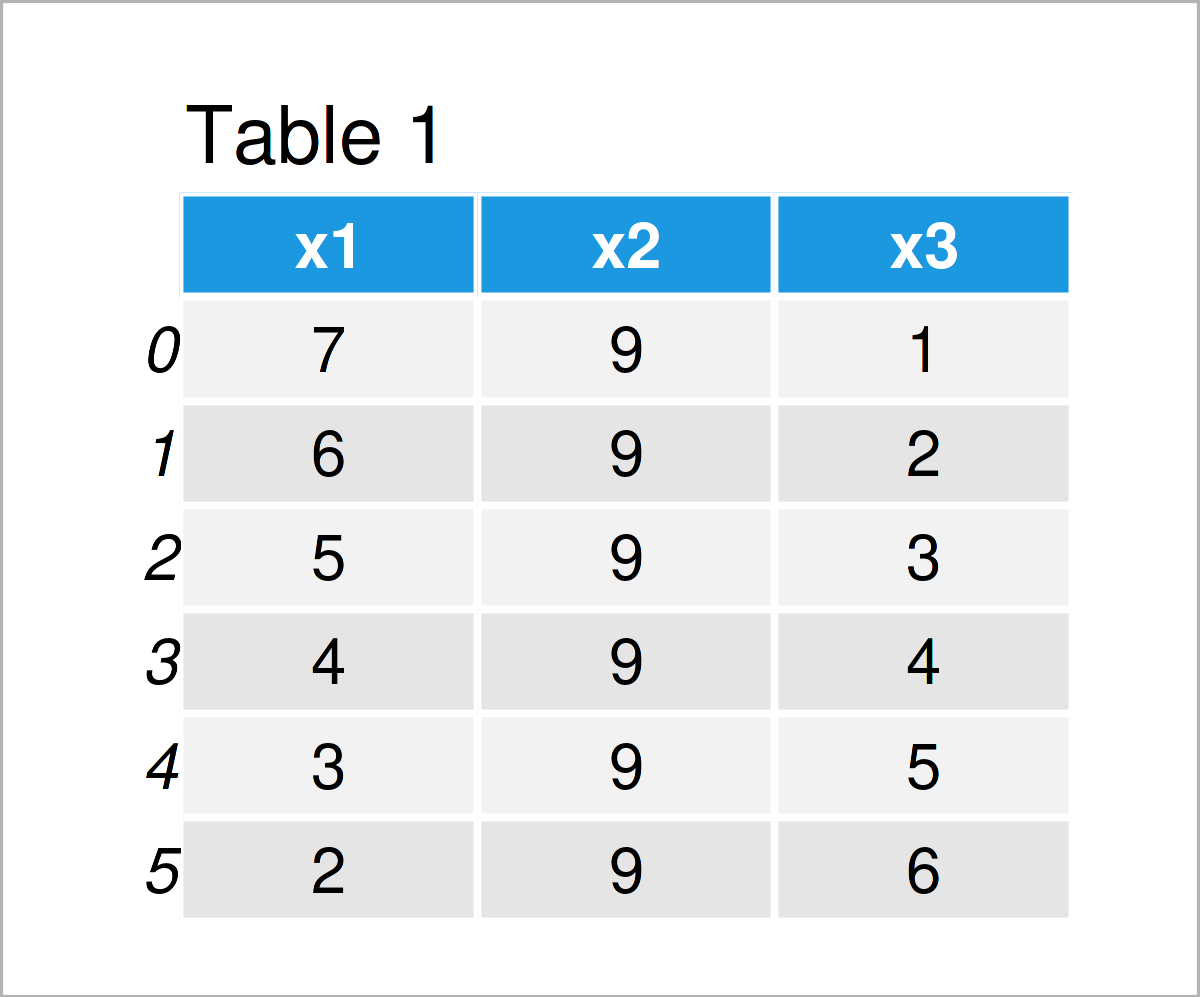# Get Values of First Row in pandas DataFrame in Python (2 Examples)

This article demonstrates how to extract the values of the first row of a pandas DataFrame in the Python programming language.

The tutorial will consist of two examples for the extraction of the values of the first row of a pandas DataFrame. To be more specific, the article consists of this information:

Here’s how to do it:

## Example Data & Add-On Libraries

First, we need to import the pandas library:

`import pandas as pd                             # Import pandas library to Python`

Next, I’ll also have to create some example data:

```data = pd.DataFrame({'x1':range(7, 1, - 1),    # Create example DataFrame
'x2':[9, 9, 9, 9, 9, 9],
'x3':range(1, 7)})
print(data)                                    # Print example DataFrame```Have a look at the table that got returned by the previous Python code. It shows that our example data contains six data points and the three columns “x1”, “x2”, and “x3”.

## Example 1: Return First Value of All Columns in pandas DataFrame

In this example, I’ll explain how to get the values of the very first row of a pandas DataFrame in Python.

For this task, we can use the iloc attribute of our DataFrame in combination with the index position 0.

Have a look at the following Python code:

```print(data.iloc)                            # All columns
# x1    7
# x2    9
# x3    1
# Name: 0, dtype: int64```

The previous console output shows the names of our three columns and the first value stored in each of these columns.

## Example 2: Return First Value of One Specific Column in pandas DataFrame

In this example, I’ll explain how to extract the first value of a particular variable of a pandas DataFrame.

To do this, we have to subset our data as you can see below:

```print(data['x3'].iloc)                      # Particular column
# 1```

The previous Python syntax has returned the first value of the variable x3, i.e. the value 1.

## Video, Further Resources & Summary

In case you need further info on the examples of this tutorial, I recommend having a look at the following video on my YouTube channel. In the video instructions, I’m explaining the Python programming syntax of this article in some more detail.

Please accept YouTube cookies to play this video. By accepting you will be accessing content from YouTube, a service provided by an external third party.If you accept this notice, your choice will be saved and the page will refresh.

Would you like to learn more about the extraction of the values of the first row of a pandas DataFrame? Then I recommend watching the following video, which was published on the Xperimental Learning YouTube channel.

In the video, the speaker shows how to fetch the first row of each group in a pandas DataFrame:

Please accept YouTube cookies to play this video. By accepting you will be accessing content from YouTube, a service provided by an external third party.If you accept this notice, your choice will be saved and the page will refresh.

Furthermore, you may want to have a look at the other articles on my website:

Summary: In this tutorial, I have demonstrated how to return the values of the first row of a pandas DataFrame in the Python programming language. In case you have additional questions and/or comments, please let me know in the comments.

Subscribe to the Statistics Globe Newsletter